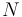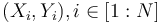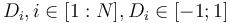# Difference between revisions of "RotorcraftMixing"

Let us consider a vehicle comprising a set of$N$ identical power trains$R_i, i \in[1:N]$ located at coordinates$(X_i,Y_i), i\in[1:N]$ and spinning in the same plane in the direction$D_i, i\in[1:N], D_i\in[-1;1]$ at a rotational speed$\omega_i, i\in[1:N]$.